# Using the quadratic formula to find n^(th) term of a sequence - consistent difference between differences

## Method 1

This is the easiest to remember but takes the most time to work out an answer.

We know (from the last section) that: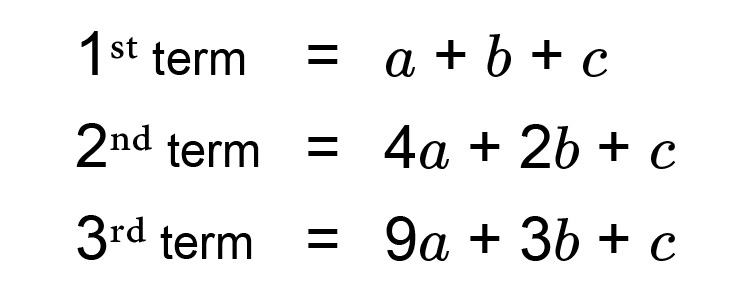Once you have found a,b,\ &\ c you can slot these figures in the following formula.

an^2+bn+c

Example 1

### Square numbers

We know the following sequence is a quadratic sequence but what is the formula for the n^(th)  term and what is the 14^(th) term?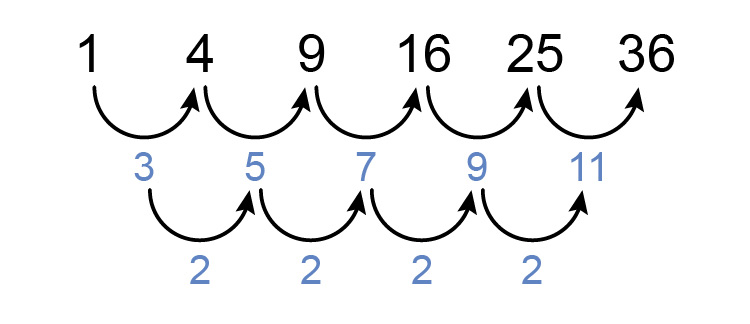The 1st term =a+b+c

so                 1=a+b+c

The 2nd term =4a+2b+c

so                   4=4a+2b+c

The 3rd term =9a+3b+c

so                   9=9a+3b+c

Now you have 3 simultaneous equations.

1=a+b+c  ....................  (1)

4=4a+2b+c  ................  (2)

9=9a+3b+c  ................  (3)

Now solve for one letter at a time

1=a+b+c

therefore     c=1-a-b

Substitute this into equations (2) and (3).

4=4a+2b+1-a-b

9=9a+3b+1-a-b

These two simultaneous equations become

4-1=4a-a+2b-b

and               9-1=9a-a+3b-b

Which simplify to

3=3a+b   ........... (i)

and  8=8a+2b   .......... (ii)

Now we can solve these two simultaneous equations

3=3a+b

is the same as b=3-3a

substitute this into (ii)

8=8a+2(3-3a)

which is         8=8a+6-6a

which is 8-6=8a-6a

becomes       2=2a

therefore      a=1

Sustitute a=1 into (i)

and          3=3a+b

becomes 3=3times1+b

b=3-3=0

Now substitute a=1 and b=0 into equation (1)

1=a+b+c

1=1+0+c

c=1-1=0

Summary a=1   b=0   and   c=0

therefore the formula for the n^(th)  term in this sequence is

an^2+bn+c

or   1n^2+0n+0

which is        n^2

Now we need to check the formula is correct.

Try different values of n in the formula n^2

If  n=1       term  =1^2=1

If  n=2       term  =2^2=4

If  n=3       term  =3^2=9

If  n=4       term  =4^2=16

This is correct

The 14th term would be

If  n=14       then  n^2=14^2=196

The 14th term =196

Example 2

We know the following sequence is a quadratic sequence but what is the formula of the n^(th) term and what is the 16th term?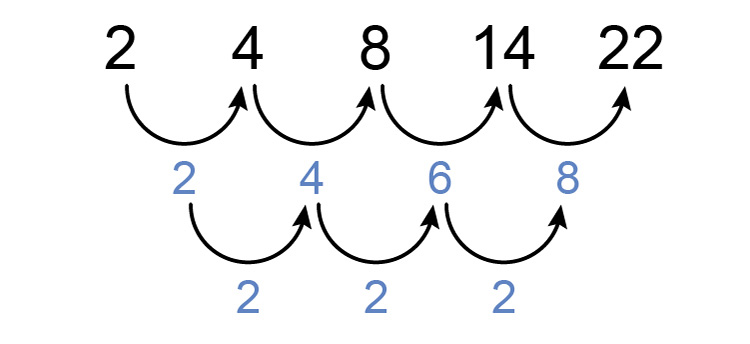The 1st term =a+b+c

so                 2=a+b+c

The 2nd term =4a+2b+c

so                   4=4a+2b+c

The 3rd term =9a+3b+c

so                  8=9a+3b+c

Now you have 3 simultaneous equations.

2=a+b+c  ....................  (1)

4=4a+2b+c  ................  (2)

8=9a+3b+c  ................  (3)

Now solve for one letter at a time

2=a+b+c

therefore     c=2-a-b

Substitute this into equations (2) and (3).

4=4a+2b+(2-a-b)

8=9a+3b+(2-a-b)

These two simultaneous equations become

4-2=4a-a+2b-b

and               8-2=9a-a+3b-b

Which simplify to

2=3a+b   ............ (i)

and  6=8a+2b   .......... (ii)

Now we can solve these two simultaneous equations

2=3a+b

is the same as b=2-3a

substitute this into (ii)

6=8a+2(2-3a)

which is         6=8a+4-6a

which is 6-4=8a-6a

becomes       2=2a

therefore      a=1

Sustitute a=1 into (i)

and          2=3a+b

becomes 2=3times1+b

b=2-3=-1

Now substitute a=1 and b=-1 into equation 1

2=a+b+c

2=1-1+c

c=2

Summary a=1   b=-1   and   c=2

therefore the formula for the n^(th)  term in this sequence is

an^2+bn+c

or   1n^2+(-1)n+2

which is        n^2-n+2

Now we need to check the formula is correct.

Try different values of n in the formula n^2-n+2

If  n=1       term  =1^2-1+2=2

If  n=2       term  =2^2-2+2=4-2+2=4

If  n=3       term  =3^2-3+2=9-3+2=8

If  n=4       term  =4^2-4+2=16-4+2=14

This is correct

The 16th term would be

If  n=16       then  n^2-n+2=16^2-16+2=256-16+2=242

The 16th term =242

Example 3

We know the following sequence is a quadratic sequence but what is the formula of the n^(th) term and what is the 12th term?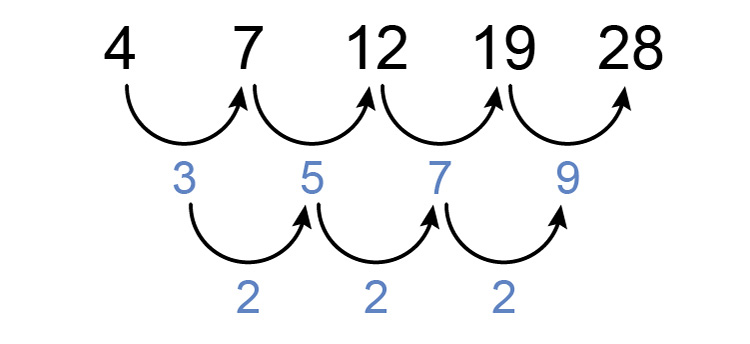The 1st term =a+b+c

so                  4=a+b+c

The 2nd term =4a+2b+c

so                   7=4a+2b+c

The 3rd term =9a+3b+c

so                12=9a+3b+c

Now you have 3 simultaneous equations.

4=a+b+c  .....................  (1)

7=4a+2b+c  ................  (2)

12=9a+3b+c  ................  (3)

Now solve for one letter at a time

4=a+b+c

therefore     c=4-a-b

Substitute this into equations (2) and (3).

7=4a+2b+(4-a-b)

12=9a+3b+(4-a-b)

These two simultaneous equations become

7-4=4a-a+2b-b

and               12-4=9a-a+3b-b

Which simplify to

3=3a+b   ........... (i)

and  8=8a+2b   .......... (ii)

Now we can solve these two simultaneous equations

3=3a+b

is the same as b=3-3a

substitute this into (ii)

8=8a+2(3-3a)

which is         8=8a+6-6a

which is 8-6=8a-6a

becomes       2=2a

therefore      a=2/2=1

Sustitute a=1 into (i)

and          3=3a+b

becomes 3=3times1+b

b=3-3=0

Now substitute a=1 and b=0 into equation (1)

4=a+b+c

4=1+0+c

c=4-1

c=3

Summary a=1   b=0   and   c=3

therefore the formula for the n^(th)  term in this sequence is

an^2+bn+c

or    1n^2+0n+3

which is          n^2+3

Now we need to check the formula is correct.

Try different values of n in the formula n^2+3

If  n=1       term  =1^2+3=1+3=4

If  n=2       term  =2^2+3=4+3=7

If  n=3       term  =3^2+3=9+3=12

If  n=4       term  =4^2+3=16+3=19

This is correct

The 12th term would be

If  n=12       then  n^2+3=12^2+3

=144+3

=147

The 12th term =147

Example 4

We know the following sequence is a quadratic sequence but what is the formula of the n^(th) term and what is the 11th term?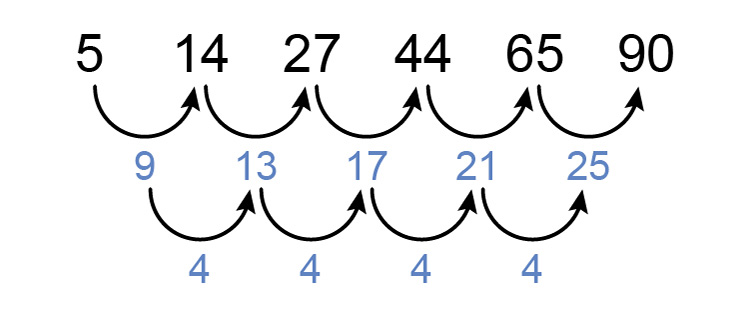The 1st term =a+b+c

so                 5=a+b+c

The 2nd term =4a+2b+c

so                 14=4a+2b+c

The 3rd term =9a+3b+c

so                27=9a+3b+c

Now you have 3 simultaneous equations.

5=a+b+c  ....................   (1)

14=4a+2b+c  ................  (2)

27=9a+3b+c  ................  (3)

Now solve for one letter at a time

5=a+b+c

therefore     c=5-a-b

Substitute this into equations (2) and (3).

14=4a+2b+5-a-b

27=9a+3b+5-a-b

These two simultaneous equations become

14-5=4a-a+2b-b

and               27-5=9a-a+3b-b

Which simplify to

9=3a+b   ............ (i)

and  22=8a+2b   .......... (ii)

Now we can solve these two simultaneous equations

9=3a+b

is the same as b=9-3a

substitute this into (ii)

22=8a+2(9-3a)

which is            22=8a+18-6a

which is 22-18=8a-6a

becomes            4=2a

therefore           a=4/2=2

Sustitute a=2 into (i)

and          9=3a+b

becomes 9=3times2+b

b=9-6=3

Now substitute a=2 and b=3 into equation (1)

5=a+b+c

5=2+3+c

c=0

Summary a=2   b=3   and   c=0

therefore the formula for the n^(th)  term in this sequence is

an^2+bn+c

or    2n^2+3n+0

which is        2n^2+3n

Now we need to check the formula is correct

Try different values of n in the formula 2n^2+3n

If  n=1       term  =2times1^2+3times1=2+3=5

If  n=2       term  =2times2^2+3times2=8+6=14

If  n=3       term  =2times3^2+3times3=18+9=27

If  n=4       term  =2times4^2+3times4=32+12=44

This is correct

The 11th term would be

If  n=11       then  2n^2+3n=2times11^2+3times11=242+33=275

The 11th term =275